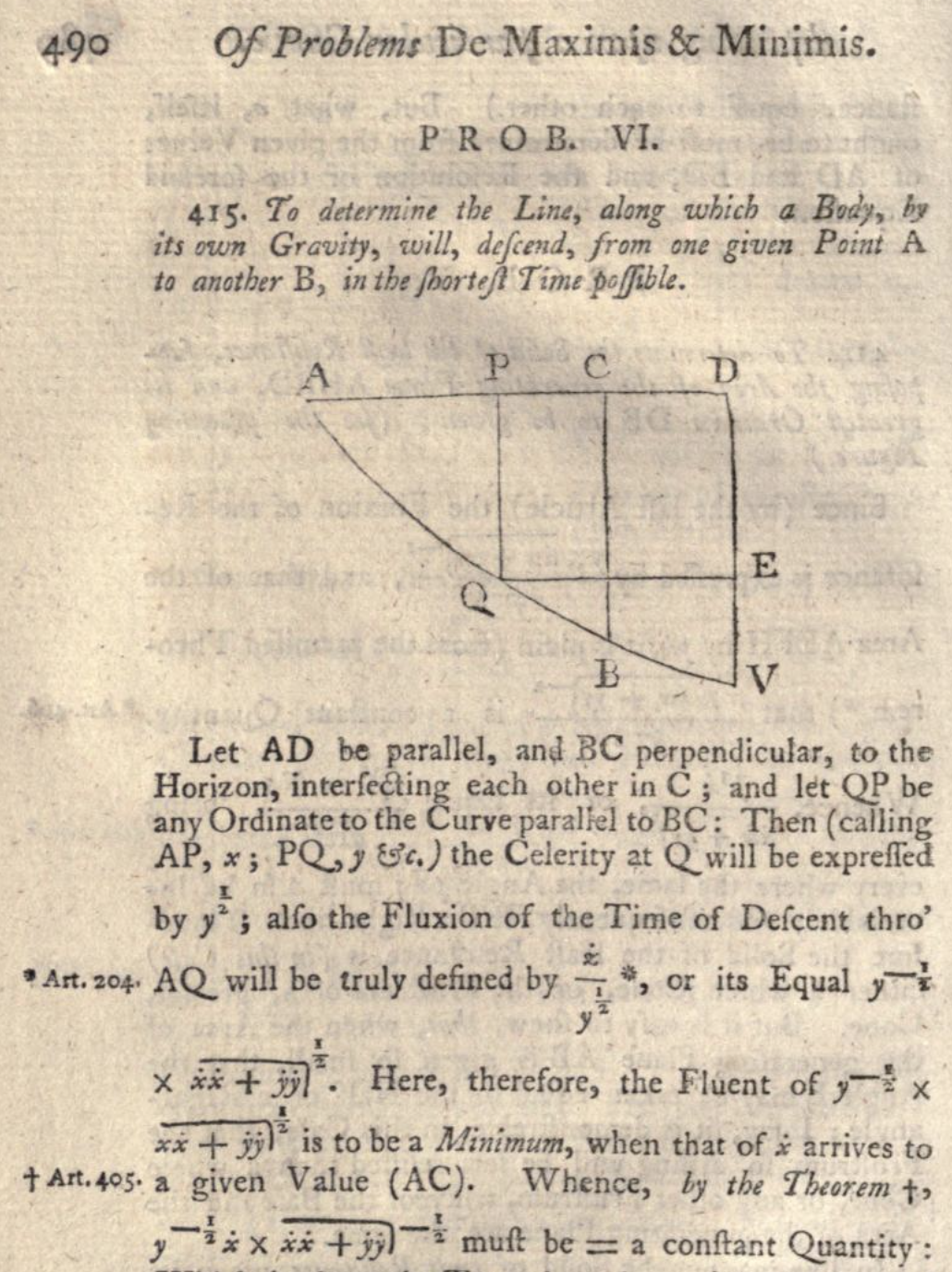# Reference request: Oldest calculus, real analysis books with exercises?

Per the title, what are some of the oldest calculus, real analysis books out there with exercises? Maybe there are some hidden gems from before the 20th century out there.

Edit. Unsolved exercises are fine. Same with solved.

It depends if you mean exercises with or without solutions.

The former don’t differ much from example-driven textbooks (e.g. Euler’s Institutiones (1755, 1768, 1769, 1770), Lehmus’ Uebungs-Aufgaben (1823), Gregory’s Examples (1841), Sohncke’s Aufgaben (1850), Todhunter’s Treatise (1852, 1857), Lübsen’s Selbstunterricht books (1853-), Schlömilch’s Übungsbücher (1868, 1870)), papers (e.g. Cauchy’s Exercices (1826-) are really reprints of his papers), or tables (e.g. Bierens de Haan (1858)). In fact, like many “earliest” questions this soon devolves into meaninglessness, as old texts often called Proposition what we would call an Exercise or Problem: e.g. Newton (1687), l’Hôpital (1696) — in that sense they all qualify.

The latter are a more recent phenomenon, e.g. Boole’s book (1859) seems to be an early one with mostly unsolved exercises at chapters’ ends.

In my search for "the oldest", I found two pre-1800 textbooks on analysis with solved problems:

Select Exercises for Young Proficients in the Mathematicks is from 1752. Part 5 deals with the method of fluxions, which is how Newton referred to real analysis.

More solved problems on fluxions by the same author, Thomas Simpson, are in his 1776 book The doctrine and application of fluxions.

Here is one problem from the 1776 book:L'hôpital's 1696 Analyse des Infiniment Petits was the most popular and influential calculus textbook of the eighteenth century. Here is an image from plate 7 in John Quincy Adams's copy:This corresponds to the following problem from page 201:

Soient données trois lignes quelconques L, M, N; (Fig. 128, Pl. 7) & soient entendues de chacun des points L, l de la ligne L deux tangents LM & LN, lm & ln, aux deux courbes M & N, une à chacune. On demande la quatriéme courbe C, qui ait pour tangentes toutes les droites MN, mn qui joignent les points touchans des courbes M, N.

I translate this as:

Let three curves $$L, M, N$$ be given, and from each point $$l$$ on the curve $$L$$ let the two tangents $$lm$$ and $$ln$$ be extended to the two curves $$M$$ and $$N$$. What is the fourth curve $$C$$, which has for tangents all the lines $$mn$$ which join the points touching the curves $$M$$ and $$N$$?

• There is an annotated english traslation of the same here by Springer. – user57432 Oct 27 '18 at 3:34

Maria Agnesi is perhaps best (only?) known for the "witch of Agnesi" today, but her calculus textbook has much more than that to recommend it. I'm not sure if it contains "exercises" in your sense of the word, though.

• For the curious, there are multiple scans of "Instituzioni analitiche ad uso della gioventù italiana" on the internet archive: e.g. archive.org/details/bub_gb_QN8rO4MZeckC and the "Similar items" displayed on that page. – j.c. Oct 27 '18 at 21:38
• "...known for the 'witch of Agnesi'..." - and this is of course a mistranslation of "versiera". – J. M. isn't a mathematician Aug 4 '19 at 10:32

I heard that one popular real analysis textbook during beginning of 20th century is the Goursat. You can read an English translation at here:

https://archive.org/details/coursemathanalys01gourrich/page/n99